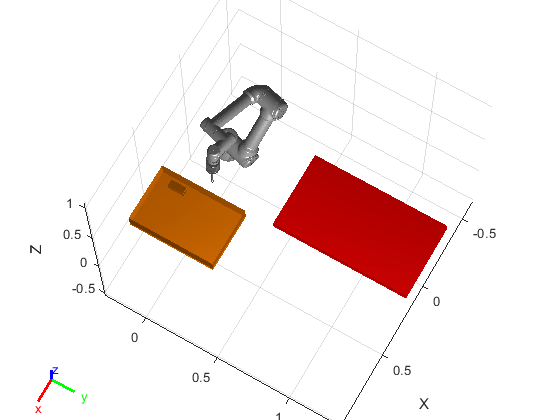# Motion Planning and RigidBodyTree Simulation of UR5e for Bin Picking Using manipulatorRRT Algorithm

This example shows the motion planning algorithm design workflow for a real-world cobot bin picking application. The rigid body tree considered in this example is for Universal Robots URe5 cobot with the added end-effector along with the suction gripper (Robotiq Vaccum Gripper - EPick).

For a real-world industrial based application, it is important to generate and execute cobot trajectories that avoid the collision with the surrounding environment (that is, design a motion planner that satisfies the collision-free trajectory requirement). This example uses `manipulatorRRT` algorithm that generates a collision-free path from the starting position to destination, and also uses the `contopptraj` algorithm to compute the fastest possible way to traverse the path while still stopping at each waypoint.

To know more about how to use manipulatorRRT algorithm for motion planning, refer to this example.

### Toolbox dependency

This example uses:

1. MATLAB

2. Robotics System Toolbox

3. Optimization Toolbox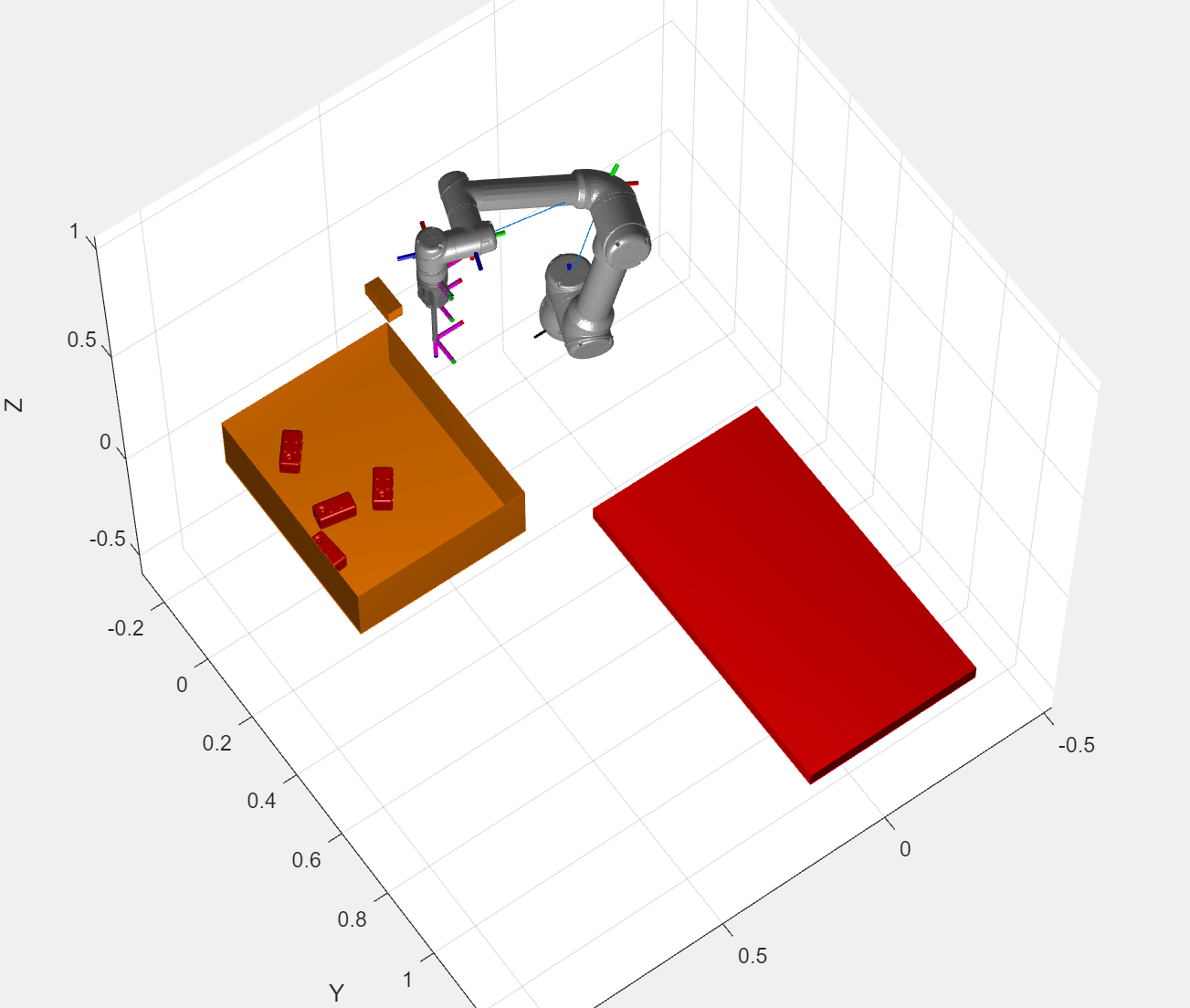### Create Environment with UR5e, Bin, and Parts

#### Load Universal Robots UR5e robot as a RigidBodyTree model

Load rigid body tree model for Universal Robot UR5e robot manipulator using `importrobot`.

`ur5eRBT = loadrobot('universalUR5e','DataFormat','row');`

#### Attach Robotiq Vaccum Gripper Body at the Robot End-effector Body

The function `exampleHelperAddGripper` is used to attach the additional end-effector body (Robotiq Vaccum Gripper - EPick) as a collision object for precise motion planning.

`ur5e = exampleHelperAddGripper(ur5eRBT);`

#### Show Robot RigidBodyTree Model at Home Position

Home position is the initial position of the robot from where we start a pick-and-place cycle. It is also possible to keep the home position same as the retract posion. if it is allowed in the environment.

These are the guidelines to select home position of the robot.

• Select home position as near to the center of the bin as possible, at some height.

• Ensure that the home position is feasible enough for generating a safe trajectory in the collision environment.

• If possible, try to select home position same as retract position (Retract position will be discussed in the further section)

```% Home Position homePosition = deg2rad([-15 -126 113 -80 -91 76]); % Set home position of each joint ur5e.Bodies{1, 3}.Joint.HomePosition = homePosition(1); ur5e.Bodies{1, 4}.Joint.HomePosition = homePosition(2); ur5e.Bodies{1, 5}.Joint.HomePosition = homePosition(3); ur5e.Bodies{1, 6}.Joint.HomePosition = homePosition(4); ur5e.Bodies{1, 7}.Joint.HomePosition = homePosition(5); ur5e.Bodies{1, 8}.Joint.HomePosition = homePosition(6); % Show robot at home position f1 = figure; show(ur5e,homePosition,'Frames','on','PreservePlot',false,'Collisions','off','Visuals','on'); hold on```#### Bin and Part dimensions

Define bin and part dimensions. This data will be used to create a collision environment for the motion planner.

Note: All dimensions are in meters.

```% bin dimension binLength = 0.38; % Along X axis binWidth = 0.57; % Along Y axis binHeight = 0.11; binCenterPosition = [0.48 0 -0.09+0.11/2]; binRotation = 0; % part dimension partheight = 0.0508; partWidth = 0.0508;```

#### Plot Bin Along with Robot RigidBodyTree Model

```env = exampleHelperPlotBin(binLength, binWidth, binHeight, binCenterPosition, binRotation); hold on```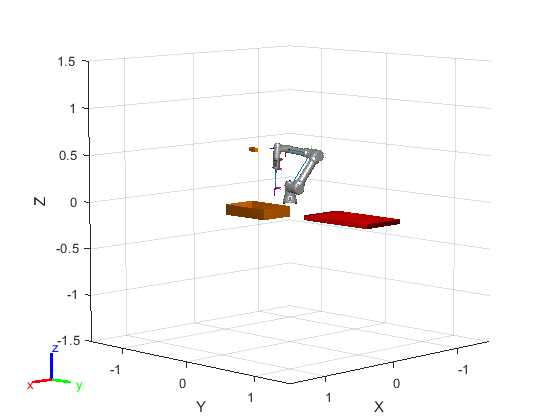#### Generate Part's Ground Truth and Plot in the Semi-Structured Enviornment

This example provides `exampleHelperGenerateGT` function to generate ground truth for the objects. Another function, `exampleHelperPlotParts `is used to visualize the parts in the bin in the semi-structured robot environment.

#### Algoritm to spawn parts

The algorithm randomizes the center location of parts in the bin, the rotation of parts with respect to the central axis, and the face on which the parts are lying. After spawning the part, it also checks for the collision with the wall or existing parts and accepts or rejects the newly spawned part accordingly.

Location of Center

The location of the center of the part is generated by randomized algorithm and it lies between the red boundary. The location of the part's center point must be in such a way that it does not collide with the bin wall or the neighbour parts.

Rotation

The rotation of the part is randomized between 0 to 360 degrees. Because the part has a distinct feature on one side, 0 and 180 degrees are not the same configurations.

Collision with bin walls and existing parts

Collision of each newly spawned part with the bin walls and existing parts in the environment is validated if the part's center is within a specified radius from the boundry or center of the other part. Each part is represented with a collision box.

The part ground truth is defined as the 1x4 array: [centerX, centerY, centerZ, rotationZ]

```% Number of parts numberOfParts = 5; % Generate part ground truth using randomization approach [partGT,env] = exampleHelperGenerateGT(binLength, binWidth, binHeight, binCenterPosition, binRotation,numberOfParts,env); % Plot Parts exampleHelperPlotParts(binLength, binWidth, binHeight, binCenterPosition, binRotation, partGT); % Adjust the camera and axis limits axis auto; view([120 70]);```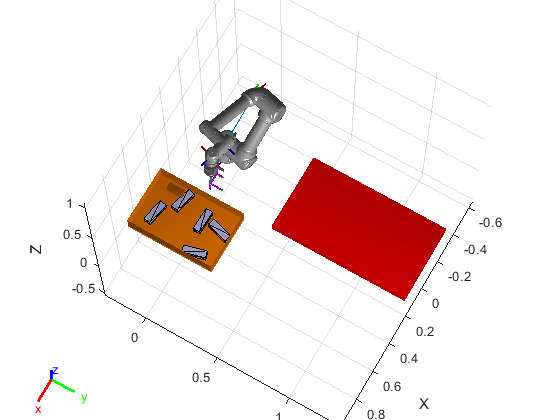```drawnow; % Reset the seed rng shuffle```

### Motion Planning

The algorithm used in the upcoming sections uses these flags that you can set to `true` or `false`.

• `showAnimation` - if set to `true`, MATLAB shows the animation of the robot traversing the path to pick and place the parts.

• `showAnimationTraj` - if set to `true`, MATLAB shows the animation of the robot traversing the path to pick and place the parts as depicted by the interpolated trajectory. This is the final trajectory that we send to the hardware.

• `isDesign` - if set to `true`, MATLAB uses workspace goal region and inverse kinematics to compute intermediate joint configurations, which results in a lower cycle time. If you set this flag to `false`, MATLAB uses hardcoded joint configurations.

```% Flag for visualization showAnimation = true; showAnimationTraj = false; % Flag for design isDesign = true; placeAngleOffset = 79.0222; % Only applicable when not using IK % Threshold for pose error poseThr = 1e-3; % Radius to switch planner planRadius1 = 0.18; planRadius2 = 0.21;```

#### Motion Planning of UR5e Cobot Using manipulatorRRT Algorithm

The overall motion planning in this example is divided into four different sections.

1. Approach

2. Retract

3. Place

4. Reset

#### Approach

The robot starts each cycle from a home position such that an easy and intuitive pick approach exists. The end-goal for this section is to reach to the part surface. The end-goal is adjusted based on the face of the part which is on the top. The goal is defined by workspace goal region with degrees of freedom (DoFs) on Z axis.#### Retract

The retract motion lifts the part above the walls of the bin. This section of motion planning helps the RRT-based planner to converge more reliably during the next phase of planning. The workspace goal region is removed for the retract position and we provide fixed joint configuration as the goal to the planner to make it more computationally efficient and reduce cycle time. However, you can remove this part of planning if it is not required or if it does not result in additional benefit.During the planning process, the robot collision model is also modified to include the part attached at the end of the gripper. If this is not done, the planner might give a trajectory that may result in the part hitting the walls of the bin, even though the gripper does not hit the walls.

#### Place

If you know the place configuration, you can remove inverse kinematics solver and use preconfigured joint configurations instead, along with modifying the last joint based on the part's orientation. This reduces the computation time and cycle time.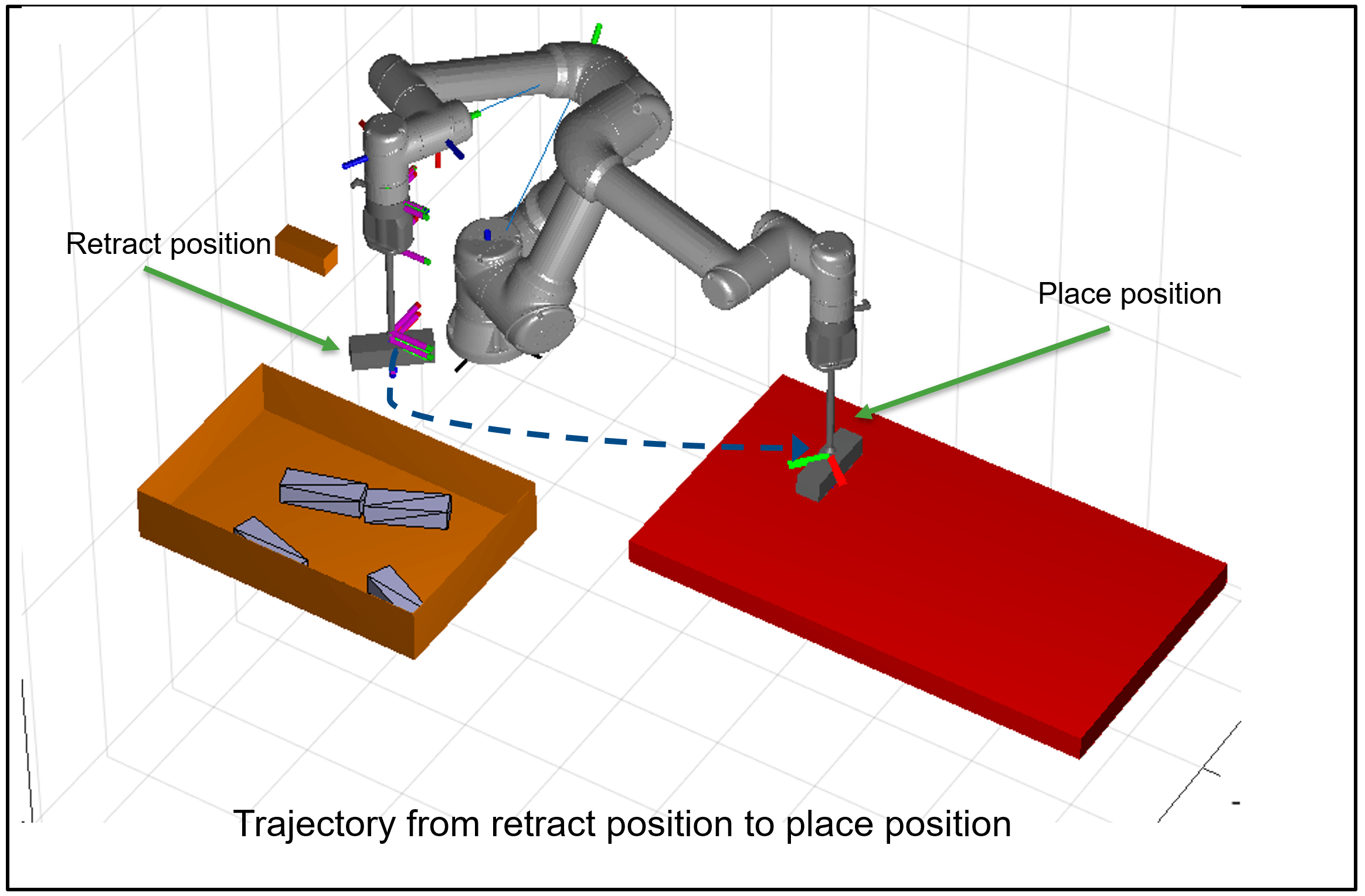Note: It is important to give a good initial guess to the inverse kinematics solver. Because we know the approximate drop location, it is relatively easy to come up with a very close initial guess for the drop location.

#### Reset

This motion allows hard reset of all joint angles to the preferred range before starting the next cycle. It helps in keeping the motion of the robot predictable and reliable.#### Part Selection algorithm

From the available parts to pick, the algorithm calculates distance between center of the part and the center of the bin. The part with the least distance from the bin's center is selected for the next pick-place cycle.Scheduling of Planner Parameters

• Blue : Cluttered environment, move carefully

• Green : Less cluttered environment, can have bigger steps

• Grey : Almost empty environment, motion is highly restricted, take large steps towards centerTrajectory Generation Using contopptraj

The final section of the planner uses the interpolated plan to generate q, qd and qdd commands for the real-world use case. The motion is divided into three sections.

1. Home to Pick - Trajectory Control

2. Pick to Place - Trajectory Control

3. Place to Home - Joint angle Control

The trajectories are generated using `contopptraj`, which provides constrained time optimal trajectories. The `Pick to Place` trajectory is generated by combining two separate plans. Due to this, the commanded end-effector velocity has a certain dip at the retract location. In the current implementation, this is handled by providing a normalized time allocation to `Pick to Retract` and `Retract to Place` based on the distance in joint space, and using this as the initial time guess to formulate piecewise polynomial with spline interpolation.

#### Motion Planning and Goal Execution

Start planning for the pick and place of the each object one by one.

`RemainingParts = numberOfParts;`

Create a goal frame object for further visualization.

`goalFrame = robotics.manip.internal.workspaceGoalRegion.FrameVisual;`

Run a loop for each available parts.

```for p = 1:numberOfParts disp("Starting computation for approach trajectory"); %%%%%% Approach trajectory %%%%%% % Define Goal Region goalRegion = workspaceGoalRegion(ur5e.BodyNames{end}); % Add bounds for the goal region goalRegion.Bounds(1, :) = [0 0]; % X Bounds goalRegion.Bounds(2, :) = [0 0]; % Y Bounds goalRegion.Bounds(3, :) = [0 0]; % Z Bounds goalRegion.Bounds(4, :) = [0 0]; % Rotation about the Z-axis goalRegion.Bounds(5, :) = [0 0]; % Rotation about the Y-axis goalRegion.Bounds(6, :) = [0 0]; % Rotation about the X-axis % Select Part to pick using the part selection algorithm (It selects % the nearset part to the bin center point) [RefPose,partID,goalZoffset] = exampleHelperSelectPart(partGT,binCenterPosition); % Create goal region based on part position and its reference pose goalRegion.ReferencePose = trvec2tform(RefPose); goalRegion.ReferencePose(1:3,1:3) = eul2rotm([0 deg2rad(binRotation) 0]); goalRegion.EndEffectorOffsetPose = trvec2tform([0 0 goalZoffset]); goalRegion.EndEffectorOffsetPose = ... goalRegion.EndEffectorOffsetPose*eul2tform([0 pi 0],"ZYX"); % Visualize the goal region for the approach figure(f1); hold on show(goalRegion); drawnow; % Create RRT planner using robot rigid body tree and the available % collision enviornment planner = manipulatorRRT(ur5e,env); % Set planner parameters planner.MaxConnectionDistance = 0.1; planner.ValidationDistance = 0.1; planner.SkippedSelfCollisions = 'parent'; numIter = 20; % plan approach trajectory and compute path path = plan(planner,homePosition,goalRegion); % Compute short and interpolated path using the computed path shortPath = path; interpConfigurations = interpolate(planner,shortPath); cumulativePath{1} = interpConfigurations; % Show animation of the interpolated path if showAnimation rateObj = rateControl(30); for i = 1 : size(interpConfigurations) show(ur5e,interpConfigurations(i,:),'PreservePlot',false,'Frames','off','Collisions','off','Visuals','on','FastUpdate',true); drawnow waitfor(rateObj); end end hold off endEffectorApproachTransform = getTransform(ur5e,interpConfigurations(end,:),ur5e.BodyNames{end}); eulerAtEndEffector = tform2eul(endEffectorApproachTransform); % Add part as a collision box with the robot end-effector tool ur5e = exampleHelperAttachPart(ur5e,-deg2rad(partGT(partID,4))+eulerAtEndEffector(1)+pi); % Remove part from the collision environment (remove part from the bin % as it attached to the robot end-effector tool) index = false(1,length(env)); index(7+partID) = true; env(index) = []; %%%%%% Retract trajectory %%%%%% disp("Starting computation for retract trajectory") % Update the Planner for the modified robot and collision enviornment planner = manipulatorRRT(ur5e,env); % Update the planner paramters based on the distance of the object to % the center of the bin. Below code set the planner parameter based on % the enviornment complexity to optimize the planning computation dist = norm(partGT(partID,1:2) - binCenterPosition(1:2)); shortFlag = false; if dist < planRadius1 planner.MaxConnectionDistance = 0.05; planner.ValidationDistance = planner.MaxConnectionDistance/4; elseif dist > planRadius2 planner.MaxConnectionDistance = 0.15; planner.ValidationDistance = planner.MaxConnectionDistance/2; shortFlag = true; else planner.MaxConnectionDistance = 0.1; planner.ValidationDistance = planner.MaxConnectionDistance/3; shortFlag = true; end planner.SkippedSelfCollisions='parent'; planner.IgnoreSelfCollision = true; % Compute the end pose for the retract based on the available paramters % using a goal region. If you have computed this once then you can set % "isDesign" to false and use the pre-computed configuration to save % the computation time. if isDesign % Define Goal Region goalRegion = workspaceGoalRegion(ur5e.BodyNames{end}); goalRegion.ReferencePose(1:3,1:3) = endEffectorApproachTransform(1:3,1:3); goalRegion.Bounds(1, :) = [0 0]; % X Bounds goalRegion.Bounds(2, :) = [0 0]; % Y Bounds goalRegion.Bounds(3, :) = [0 0]; % Z Bounds goalRegion.Bounds(4, :) = [0 0]; % Rotation about the Z-axis goalRegion.Bounds(5, :) = [0 0]; % Rotation about the Y-axis goalRegion.Bounds(6, :) = [0 0]; % Rotation about the X-axis goalRegion.ReferencePose(1:3,4) = [binCenterPosition(1)-0.18 binCenterPosition(2) 0.22]'; goalRegion.EndEffectorOffsetPose = trvec2tform([0 0 0]); goalRegion.EndEffectorOffsetPose = ... goalRegion.EndEffectorOffsetPose*eul2tform([0 0 0],"ZYX"); % Show goal region hold on; show(goalRegion); drawnow; % Compute path for retract based on the given goal region path = plan(planner,interpConfigurations(end,:),goalRegion); else % Pre-computed retract goal configuration goalConfigurarion = [-0.2240 -1.3443 1.2348 -1.4613 -1.5708 1.3468]; % Show goal frame goalFrame.Pose = getTransform(ur5e,goalConfigurarion,ur5e.BodyNames{end}); hold on; show(goalFrame,gca); drawnow; % Compute path for retract based on the given goal configuration path = plan(planner,interpConfigurations(end,:),goalConfigurarion); end % Compute the short and interpolated path if shortFlag shortPath = shorten(planner,path,numIter); interpConfigurations = interpolate(planner,shortPath); else shortPath = path; interpConfigurations = interpolate(planner,shortPath); end cumulativePath{2} = interpConfigurations; % Delete Goal Region from the figure delete(findobj(f1,'type', 'hgtransform')); hold on; if isDesign show(goalRegion); else show(goalFrame,gca); end % Delete the part from the figure pt = findobj(f1,'type', 'patch','Tag','part'); % Delete Patch delete(pt((RemainingParts - partID + 1))); % Show animation of the interp configuration for retract trajectory if showAnimation rateObj = rateControl(60); for i = 1 : 5 : size(interpConfigurations) show(ur5e,interpConfigurations(i,:),'PreservePlot',false,'Frames','off','Collisions','off','Visuals','on','FastUpdate',true); waitfor(rateObj); end end % Show robor last frame show(ur5e,interpConfigurations(end,:),'PreservePlot',false,'Frames','off','Collisions','off','Visuals','on','FastUpdate',true); %%%%%% Place trajectory %%%%%% % Compute end pose for the placing the object if isDesign % Fixed End-Position so using IK instead of the work spaec goal region targetPoseAngle = [-deg2rad(partGT(partID,4))+eulerAtEndEffector(1)-pi/2 pi 0]; targetPoseXYZ = [0 0.7 0.01+partheight]; targetPose = trvec2tform(targetPoseXYZ)*eul2tform(targetPoseAngle,"ZYX"); goalFrame.Pose = targetPose; % Create IK object and set paramters ik = inverseKinematics('RigidBodyTree',ur5e); ik.SolverParameters.AllowRandomRestart = false; ik.SolverParameters.GradientTolerance = 1e-13; ik.SolverParameters.MaxTime = 5; weights = [1 1 1 1 1 1]; % Weights % Set favourable initial guess initialGuess = [1.3792 -1.0782 1.2490 -1.7416 -1.5708 1.7333]; % Compute IK solution for target pose [configSoln,solnInfo] = ik(ur5e.BodyNames{end},targetPose,weights,initialGuess); % Check for pose threshold. If condition does not satisfies then % compute IK again with random restart if solnInfo.PoseErrorNorm > poseThr ik.SolverParameters.MaxTime = 10; ik.SolverParameters.AllowRandomRestart = true; [configSoln,solnInfo] = ik(ur5e.BodyNames{end},targetPose,weights,initialGuess); if solnInfo.PoseErrorNorm > poseThr warning("IK Failure"); configSoln = [0.7950 -0.5093 0.2500 -1.3115 -1.5708 0]; end planner.EnableConnectHeuristic = false; planner.SkippedSelfCollisions='parent'; planner.IgnoreSelfCollision = true; end else % Fixed joint configuration based on previous analysis configSoln = [1.3792 -1.0821 1.2291 -1.7178 -1.5708 wrapToPi(deg2rad(partGT(partID,4)+placeAngleOffset))]; goalFrame.Pose = getTransform(ur5e,configSoln,ur5e.BodyNames{end}); end % Parameters for the planner for the place trajectory planner.MaxConnectionDistance = 0.5; planner.ValidationDistance = planner.MaxConnectionDistance/2; % Show the place configuration with the robot in rigid body tree % environemnt with goal frame figure(f1); hold on show(goalFrame,gca); drawnow; % Compute path, short path and interpolated path for the place path = plan(planner,interpConfigurations(end,:),configSoln); shortPath = shorten(planner,path,numIter); interpConfigurations = interpolate(planner,shortPath); cumulativePath{3} = interpConfigurations; % Delete Goal Region from the figure delete(findobj(f1,'type', 'hgtransform')); hold on; show(goalFrame,gca); % Show animation of the interpolated configuration for place trajectory if showAnimation rateObj = rateControl(30); for i = 1 : size(interpConfigurations) show(ur5e,interpConfigurations(i,:),'PreservePlot',false,'Frames','off','Collisions','off','Visuals','on','FastUpdate',true); waitfor(rateObj); end end % Delete Part from the body after placing the object (Modify the robot % rigid body tree enviornment) removeBody(ur5e,'part'); RemainingParts = RemainingParts - 1; %%%%%% Rest position %%%%%% % Send robot to home position after placing the object % Update the Planner planner = manipulatorRRT(ur5e,env); planner.MaxConnectionDistance = 1; planner.ValidationDistance = 0.5; planner.SkippedSelfCollisions = 'parent'; path = plan(planner,interpConfigurations(end,:),homePosition); shortPath = shorten(planner,path,numIter); cumulativePath{4} = shortPath; interpConfigurations = interpolate(planner,shortPath); % Delete Robot from the figure delete(findobj(f1,'type', 'hgtransform')); if showAnimation rateObj = rateControl(60); for i = 1 : size(interpConfigurations) show(ur5e,interpConfigurations(i,:),'PreservePlot',false,'Frames','off','Collisions','off','Visuals','on','FastUpdate',true); waitfor(rateObj); end end %% Trajectory interpolation using contopptraj with the max acceleration and max velocity bounds % Robot Parameters maxqd = pi/2; % in rad/s maxqdd = deg2rad(100); % in rad/s2 vellimits = repmat([-maxqd; maxqd],1,6); accellimits = repmat([-maxqdd; maxqdd],1,6); % Interpolate the approach trajectorie path1 = cumulativePath{1}; [q,qd,qdd,t] = contopptraj(path1',vellimits',accellimits',NumSamples=size(path1,1)*10); % Show animation of final interpolated trajectory if flag is enable if showAnimationTraj hold on; rateObj = rateControl(30); for i = 1 : size(q,2) show(ur5e,q(:,i)','PreservePlot',false,'Frames','off','Collisions','off','Visuals','on','FastUpdate',true); waitfor(rateObj); end end % Interpolate retract + place trajectory (Note that this is combine % trajectory) path21 = cumulativePath{2}; path22 = cumulativePath{3}; % Normalised distance in joint space dist_joints_1 = norm(cumulativePath{2}(1,:)-cumulativePath{2}(end,:)); dist_joints_2 = norm(cumulativePath{3}(1,:)-cumulativePath{3}(end,:)); dist_total = dist_joints_1 + dist_joints_2; % Combine both trajectory path2=[path21;path22]; initialGuessPath2 = [linspace(0,dist_joints_1/dist_total,size(path21,1)) linspace(dist_joints_1/dist_total,1,size(path22,1))]; % Remove Duplicate Rows path2(size(path21,1),:) = []; initialGuessPath2(size(path21,1)) = []; % Compute piece wise polynomial pp = interp1(initialGuessPath2,path2,'spline','pp'); % Apply contopptraj for retract + place trajectory [q,qd,qdd,t] = contopptraj(pp,vellimits',accellimits',NumSamples=size(path2,1)*10); % Show animation of the trajectory if showAnimationTraj hold on; rateObj = rateControl(30); for i = 1 : size(q,2) show(ur5e,q(:,i)','PreservePlot',false,'Frames','off','Collisions','off','Visuals','on','FastUpdate',true); waitfor(rateObj); end end % Delete Part Ground Truth partGT(partID,:) = []; end```
```Starting computation for approach trajectory ```
```Starting computation for retract trajectory ```
```Starting computation for approach trajectory ```
```Starting computation for retract trajectory ```
```Starting computation for approach trajectory ```
```Starting computation for retract trajectory ```
```Starting computation for approach trajectory ```
```Starting computation for retract trajectory ```
```Starting computation for approach trajectory ```
```Starting computation for retract trajectory ```